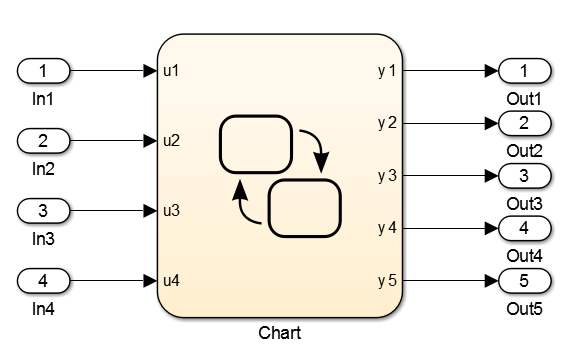Control Code Partitions for MATLAB Functions in Stateflow Charts

Simulink® PLC Coder™ inlines MATLAB® functions in generated code based on your inlining specifications. To specify whether to inline a function:

1. Right-click the MATLAB function and select Properties.

2. For Function Inline Option, select Inline if you want the function to be inlined. Select Function if you do not want the function to be inlined. For more information, see Specify Properties of MATLAB Functions (Stateflow).

• If a MATLAB function accesses data that is local to the chart, it is inlined in generated code even if you specify that the function must not be inlined.

Explanation: The chart is converted to a function block in generated code. If the MATLAB function in the chart is converted to a Structured Text function, it cannot access the data of an instance of the function block. Therefore, the MATLAB function cannot be converted to a Structured Text function in generated code and is inlined.

• If a MATLAB function has multiple outputs and you specify that the function must not be inlined, it is converted to a function block in generated code.

Explanation: A Structured Text function cannot have multiple outputs, therefore the MATLAB function cannot be converted to a Structured Text function.

The following simple example illustrates the different cases. The model used here has a Stateflow® chart that contains four MATLAB functions fcn1 to fcn4.

Here is the model.Here is the Stateflow chart.The functions fcn1 to fcn4 are defined as follows.

FunctionInlining SpecificationGenerated Code

fcn1:

function y = fcn1(u)
y = u+1;
Specify that the function must be inlined.

fcn1 is inlined in the generated code.

is_c3_Chart := Chart_IN_A;
(* Outport: '<Root>/y1' incorporates:
*  Inport: '<Root>/u1' *)
(* Entry 'A': '<S1>:10' *)
(* MATLAB Function 'fcn1': '<S1>:1' *)
(* '<S1>:1:3' *)
y1 := u1 + 1.0;

fcn2:

function y = fcn2(u)
y = u+2;
Specify that the function must not be inlined.

fcn2 is not inlined in the generated code.

is_c3_Chart := Chart_IN_B;
(* Outport: '<Root>/y2' incorporates:
*  Inport: '<Root>/u2' *)
(* Entry 'B': '<S1>:11' *)
y2 := fcn2(u := u2);
.
.
.
FUNCTION fcn2: LREAL
VAR_INPUT
u: LREAL;
END_VAR
VAR_TEMP
END_VAR
(* MATLAB Function 'fcn2': '<S1>:4' *)
(* '<S1>:4:3' *)
fcn2 := u + 2.0;
END_FUNCTION

fcn3:

function y = fcn3(u)
% The function accesses
% local data x of parent chart
y = u+3+x;
Specify that the function must not be inlined.

fcn3 is inlined in the generated code because it accesses local data from the Stateflow chart.

is_c3_Chart := Chart_IN_C;
(* Outport: '<Root>/y3' incorporates:
*  Inport: '<Root>/u3' *)
(* Entry 'C': '<S1>:15' *)
(* MATLAB Function 'fcn3': '<S1>:9' *)
(* The function accesses local data x of parent chart *)
(* '<S1>:9:4' *)
y3 := (u3 + 3.0) + x;

fcn4:

function [yy1,yy2] = fcn4(u)
yy1 = u+4;
yy2 = u+5;
Specify that the function must not be inlined.

fcn4 is converted to a function block in the generated code because it has multiple outputs.

is_c3_Chart := Chart_IN_D;
(* Entry 'D': '<S1>:28' *)
i0_fcn4(u := u4);
b_y4 := i0_fcn4.yy1;
b_y5 := i0_fcn4.yy2;
(* Outport: '<Root>/y4' incorporates:
*  Inport: '<Root>/u4' *)
y4 := b_y4;
(* Outport: '<Root>/y5' *)
y5 := b_y5;
.
.
.
FUNCTION_BLOCK fcn4
VAR_INPUT
u: LREAL;
END_VAR
VAR_OUTPUT
yy1: LREAL;
yy2: LREAL;
END_VAR
VAR
END_VAR
VAR_TEMP
END_VAR
(* MATLAB Function 'fcn4': '<S1>:26' *)
(* '<S1>:26:3' *)
yy1 := u + 4.0;
(* '<S1>:26:4' *)
yy2 := u + 5.0;
END_FUNCTION_BLOCK